## visdat

Preliminary Exploratory Visualisation of Data.

# How to install

visdat is available on CRAN

``````
install.packages("visdat")
``````

If you would like to use the development version, install from github
with:

``````
# install.packages("devtools")
devtools::install_github("ropensci/visdat")
``````

# What does visdat do?

Initially inspired by
`csv-fingerprint`,
`vis_dat` helps you visualise a dataframe and “get a look at the data”
by displaying the variable classes in a dataframe as a plot with
`vis_dat`, and getting a brief look into missing data patterns using
`vis_miss`.

`visdat` has 6 functions:

• `vis_dat()` visualises a dataframe showing you what the classes of
the columns are, and also displaying the missing data.

• `vis_miss()` visualises just the missing data, and allows for
missingness to be clustered and columns rearranged. `vis_miss()` is
similar to `missing.pattern.plot` from the
`mi`
package. Unfortunately `missing.pattern.plot` is no longer in the
`mi` package (as of 14/02/2016).

• `vis_compare()` visualise differences between two dataframes of the
same dimensions

• `vis_expect()` visualise where certain conditions hold true in your
data

• `vis_cor()` visualise the correlation of variables in a nice heatmap

• `vis_guess()` visualise the individual class of earch value in your
data

You can read more about visdat in the vignette, “using
visdat”
.

Please note that this project is released with a Contributor Code of
Conduct. By participating in this project you agree to
abide by its terms.

# Examples

## Using `vis_dat()`

Let’s see what’s inside the `airquality` dataset from base R, which
contains information about daily air quality measurements in New York
from May to September 1973. More information about the dataset can be
found with `?airquality`.

``````
library(visdat)

vis_dat(airquality)
``````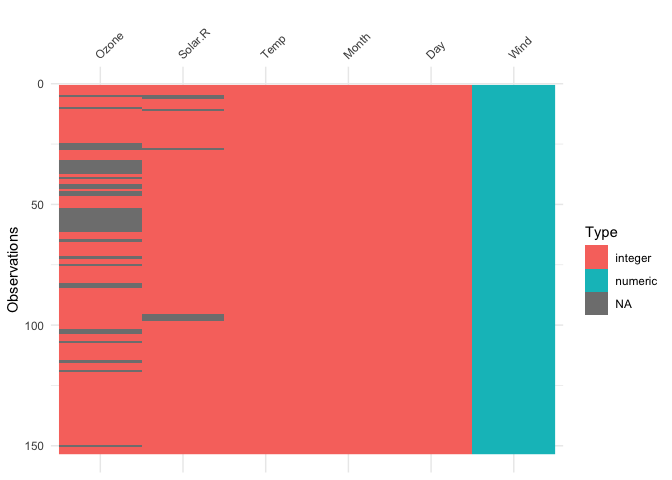The plot above tells us that R reads this dataset as having numeric and
integer values, with some missing data in `Ozone` and `Solar.R`. The
classes are represented on the legend, and missing data represented by
grey. The column/variable names are listed on the x axis.

## Using `vis_miss()`

We can explore the missing data further using `vis_miss()`:

``````
vis_miss(airquality)
``````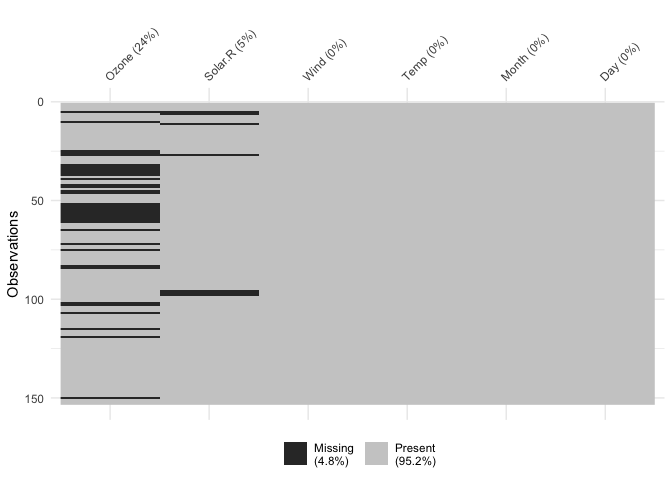Percentages of missing/complete in `vis_miss` are accurate to 1 decimal
place.

You can cluster the missingness by setting `cluster = TRUE`:

``````
vis_miss(airquality,
cluster = TRUE)
``````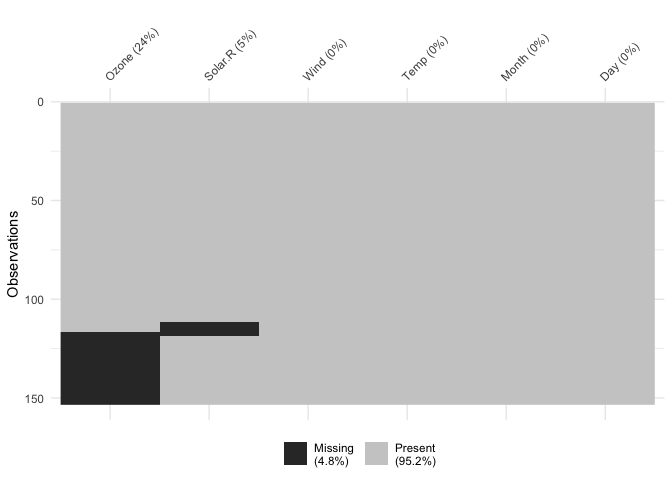Columns can also be arranged by columns with most missingness, by
setting `sort_miss = TRUE`:

``````
vis_miss(airquality,
sort_miss = TRUE)
```````vis_miss` indicates when there is a very small amount of missing data
at <0.1% missingness:

``````
test_miss_df <- data.frame(x1 = 1:10000,
x2 = rep("A", 10000),
x3 = c(rep(1L, 9999), NA))

vis_miss(test_miss_df)
``````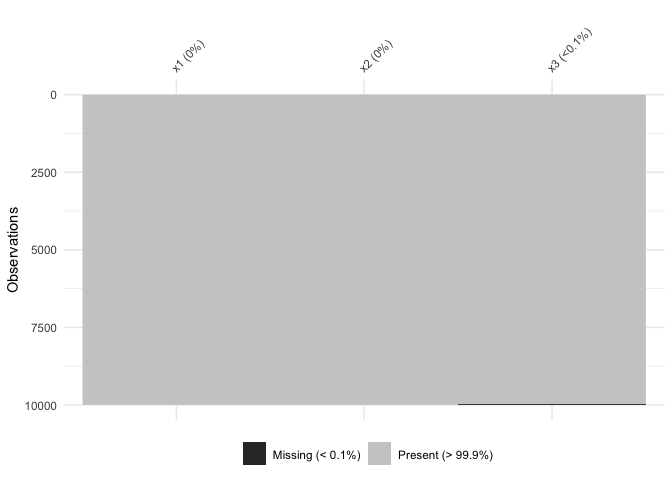`vis_miss` will also indicate when there is no missing data at all:

``````
vis_miss(mtcars)
``````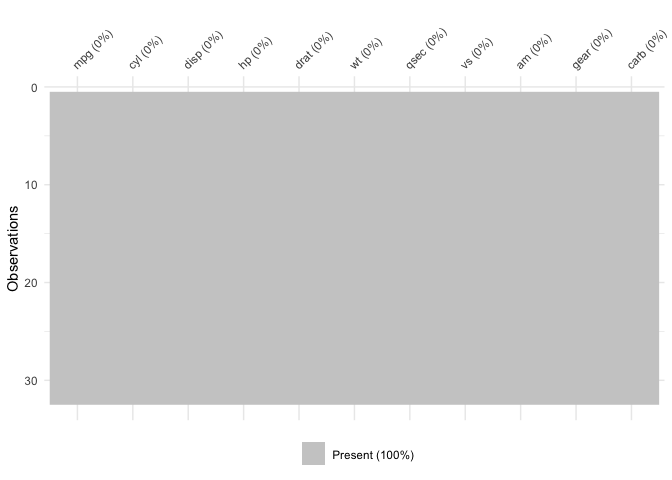To further explore the missingness structure in a dataset, I recommend
the `naniar` package, which
provides more general tools for graphical and numerical exploration of
missing values.

## Using `vis_compare()`

Sometimes you want to see what has changed in your data. `vis_compare()`
displays the differences in two dataframes of the same size. Let’s look
at an example.

Let’s make some changes to the `chickwts`, and compare this new dataset:

``````set.seed(2019-04-03-1105)
chickwts_diff <- chickwts
chickwts_diff[sample(1:nrow(chickwts), 30),sample(1:ncol(chickwts), 2)] <- NA

vis_compare(chickwts_diff, chickwts)
``````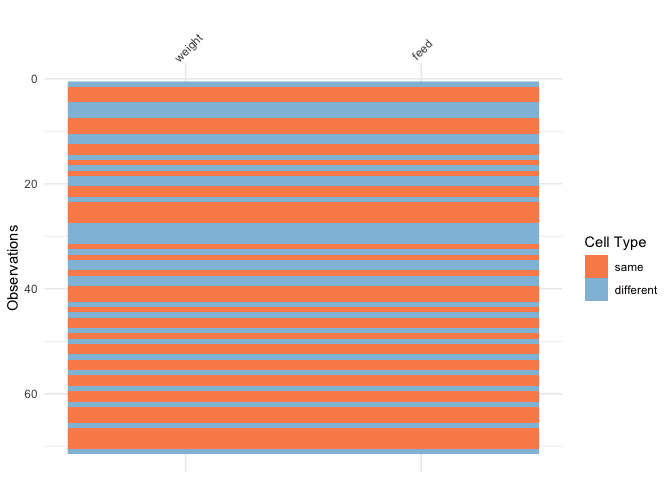Here the differences are marked in blue.

If you try and compare differences when the dimensions are different,
you get an ugly error:

``````
chickwts_diff_2 <- chickwts
chickwts_diff_2\$new_col <- chickwts_diff_2\$weight*2

vis_compare(chickwts, chickwts_diff_2)
# Error in vis_compare(chickwts, chickwts_diff_2) :
#   Dimensions of df1 and df2 are not the same. vis_compare requires dataframes of identical dimensions.
``````

## Using `vis_expect()`

`vis_expect` visualises certain conditions or values in your data. For
example, If you are not sure whether to expect values greater than 25 in
your data (airquality), you could write: `vis_expect(airquality, ~.x

= 25)`, and you can see if there are times where the values in your
data are greater than or equal to 25:

``````
vis_expect(airquality, ~.x >= 25)
``````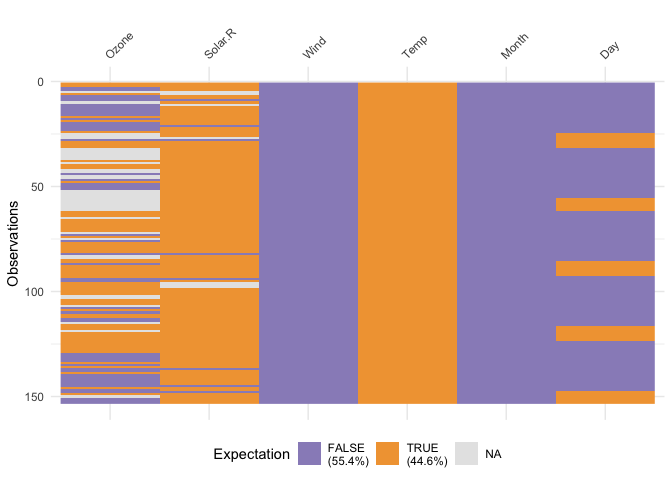This shows the proportion of times that there are values greater than
25, as well as the missings.

## Using `vis_cor()`

To make it easy to plot correlations of your data, use `vis_cor`:

``````
vis_cor(airquality)
``````## Using `vis_value`

`vis_value()` visualises the values of your data on a 0 to 1 scale.

``````vis_value(airquality)
``````It only works on numeric data, so you might get strange results if you
are using factors:

``````library(ggplot2)
vis_value(iris)
``````
``````data input can only contain numeric values, please subset the data to the numeric values you would like. dplyr::select_if(data, is.numeric) can be helpful here!
``````

So you might need to subset the data beforehand like so:

``````library(dplyr)
#>
#> Attaching package: 'dplyr'
#> The following objects are masked from 'package:stats':
#>
#>     filter, lag
#> The following objects are masked from 'package:base':
#>
#>     intersect, setdiff, setequal, union

iris %>%
select_if(is.numeric) %>%
vis_value()
``````## Using `vis_guess()`

`vis_guess()` takes a guess at what each cell is. It’s best illustrated
using some messy data, which we’ll make here:

``````
messy_vector <- c(TRUE,
T,
"TRUE",
"T",
"01/01/01",
"01/01/2001",
NA,
NaN,
"NA",
"Na",
"na",
"10",
10,
"10.1",
10.1,
"abc",
"\$%TG")

set.seed(2019-04-03-1106)
messy_df <- data.frame(var1 = messy_vector,
var2 = sample(messy_vector),
var3 = sample(messy_vector))
``````
``````
vis_guess(messy_df)
vis_dat(messy_df)
``````So here we see that there are many different kinds of data in your
dataframe. As an analyst this might be a depressing finding. We can see
this comparison above.